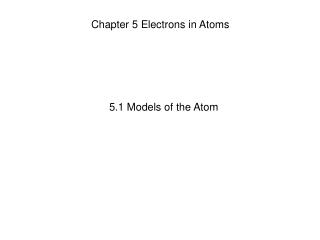DownloadDownload PresentationChapter 5 Electrons in Atoms

# Chapter 5 Electrons in Atoms

Télécharger la présentation## Chapter 5 Electrons in Atoms

- - - - - - - - - - - - - - - - - - - - - - - - - - - E N D - - - - - - - - - - - - - - - - - - - - - - - - - - -
##### Presentation Transcript

1. Chapter 5 Electrons in Atoms 5.1 Models of the Atom

2. Chemistry • Today we are learning to:-1. Realize there were problems with Rutherford’s model of the atom2. Understand the new ideas put forward in Bohr’s model of the atom • 3. Describe what is meant by a quantum mechanical model • 4. Describe what is meant by quantum energy sub levels

3. Development of Atomic Models • Rutherford’s model fails to explain why objects change color when heated. • According to Maxwell’s electromagnetic theory, this model was unstable. Electrons should spiral in towards the nucleus within a fraction of a second • A new model was needed

4. 5.1 The Development of Atomic Models Development of atomic models from 1803 to 1911.

5. 5.1 The Development of Atomic Models Development of atomic models from 1913 to 1932.

6. The Bohr Model • Niels Bohr improved on Rutherford’s model in 1913. In his model: • Each possible electron orbit has a fixed energy level. • An electron is only allowed in an energy level, not in between. • An electron can jump between levels • Higher energy levels tend to be further from the nucleus. • A quantum of energy is the amount of energy required to make an electron jump between levels.

7. The Bohr Model • Like the rungs of the strange ladder, the energy levels in an atom are not equally spaced. • The higher the energy level occupied by an electron, the less energy it takes to move from that energy level to the next higher energy level.

8. The Quantum Mechanical Model • In 1926 Erwin Schrodinger developed mathematical equations describing the behavior of an electron in a hydrogen atom • The modern description of the electrons in atoms, the quantum mechanical model, comes from the mathematical solutions to the Schrödinger equation. • Solutions give a probability of finding an electron in a certain position

9. The Quantum Mechanical Model • In 1926 Erwin Schrodinger developed mathematical equations describing the behavior of an electron in a hydrogen atom • The modern description of the electrons in atoms, the quantum mechanical model, comes from the mathematical solutions to the Schrödinger equation. • Solutions give a probability of finding an electron in a certain position

10. Atomic Orbitals • An atomic orbitalis often thought of as a region of space in which there is a high probability of finding an electron. • Principal energy levels are labelled with the quantum numbersn=1, n=2, n=3, n=4 etc. ………… • Each energy sublevel l=1, l=2, l=3, l=4, l=5, corresponds to an orbital of a different shape, which describes where the electron is likely to be found. • The sublevels are given letters to describe them. • l=1=s orbital • l=2=p orbital • l=3=d orbital • l=4=f orbital • Maximum number of electrons allowed in a principal energy level = 2n2

11. Atomic Orbitals Each energy sublevel relates to orbitals of different shape.

12. Atomic Orbitals

13. 5.1 Section Quiz. 1. Rutherford's planetary model of the atom could not explain • any properties of elements. • the chemical properties of elements. • the distribution of mass in an atom. • the distribution of positive and negative charges in an atom.

14. 5.1 Section Quiz. 2. Bohr's model of the atom proposed that electrons are found • embedded in a sphere of positive charge. • in fixed positions surrounding the nucleus. • in circular orbits at fixed distances from the nucleus. • orbiting the nucleus in a single fixed circular path.

15. 5.1 Section Quiz. 3. What is the lowest-numbered principal energy level in which porbitals are found? • 1 • 2 • 3 • 4

16. 2.3 Vocabulary 5.1Vocabulary • Energy levels:possible electron orbits in Bohr’s model of the atom • Quantum:small whole number unit of energy • Quantum mechanical model: quantum description of the movement of electrons in atoms obtained from solving Schrodinger’s equations • Atomic orbital: region of space where there is a high probability of finding an electron

17. END OF SHOW